# Supershapes (Superformula)

Written by Paul Bourke
March 2002

Based upon equations by Johan Gielis
Intended as a modelling framework for natural forms.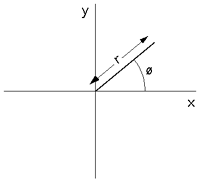The supershape equation is an extension of the both the equation of the sphere and ellipse

(x / a)2 + (y / b)2 = r2

and even the superellipse given here. The general formula for the supershape is given below.Where r and phi are polar coordinates (radius,angle). n1, n2, n3, and m are real numbers. a and b are real numbers excluding zero.

 m = 0 This results in circles, namely r = 1n1 = n2 = n3 = 1 Increasing m adds rotational symmetry to the shape. This is generally the case for other values of the n parameters. The curves are repeated in sections of the circle of angle 2π/m, this is apparent in most of the following examples for integer values of m. m=1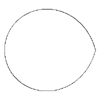m=2m=3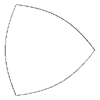m=4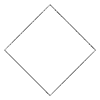m=5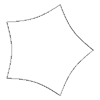m=6n1 = n2 = n3 = 0.3 As the n's are kept equal but reduced the form becomes increasingly pinched. m=1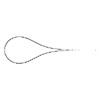m=2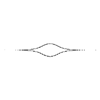m=3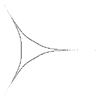m=4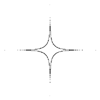m=5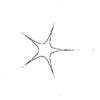m=6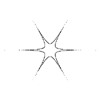If n1 is slightly larger than n2 and n3 then bloated forms result. The examples on the right have n1 = 40 and n2 = n3 = 10. m=1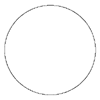m=2m=3m=4m=5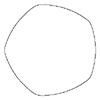m=6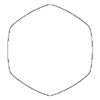Polygonal shapes are achieved with very large values of n1 and large but equal values for n2 and n3. m=3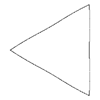m=4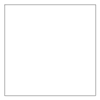m=5m=6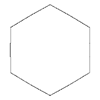Asymmetric forms can be created by using different values for the n's. The following example have n1 = 60, n2 = 55 and n3 = 30. m=3m=4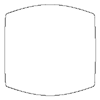m=5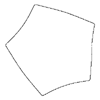m=6For non integral values of m the form is still closed for rational values. The following are example with n1 = n2 = n3 = 0.3. The angle phi needs to extend from 0 to 12π. m=1/6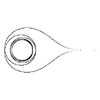m=7/6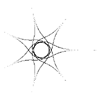m=13/6m=19/6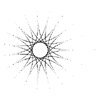Smooth starfish shapes result from smaller values of n1 than the n2 and n3. The following examples have m=5 and n2 = n3 = 1.7. n1=0.50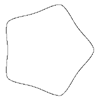n1=0.20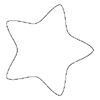n1=0.10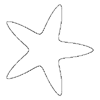n1=0.02Other examples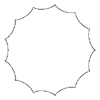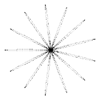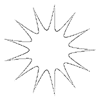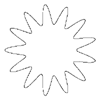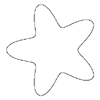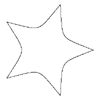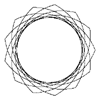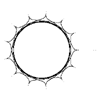Source code

Given a value of phi the following function evaluates the supershape function and calculates (x,y).

```
void Eval(double m,double n1,double n2,double n3,double phi,double *x,double *y)
{
double r;
double t1,t2;
double a=1,b=1;

t1 = cos(m * phi / 4) / a;
t1 = ABS(t1);
t1 = pow(t1,n2);

t2 = sin(m * phi / 4) / b;
t2 = ABS(t2);
t2 = pow(t2,n3);

r = pow(t1+t2,1/n1);
if (ABS(r) == 0) {
*x = 0;
*y = 0;
} else {
r = 1 / r;
*x = r * cos(phi);
*y = r * sin(phi);
}
}
```

So it might be called as follows

```   for (i=0;i<=NP;i++) {
phi = i * TWOPI / NP;
Eval(m,n1,n2,n3,phi,&x,&y);
--- do something with the point x,y ---
}
```

# Supershape in 3D

### (Also known as the Superformula)

Written by Paul Bourke
July 2003

Based upon equations by Johan Gielis
Intended as a modelling framework for natural forms.

Contribution of MSWindows version by Vincent Berthoux: supershape_win.zip

Definition of supershape in 2 dimensions.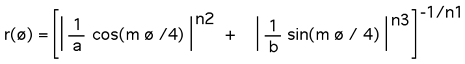Extension to 3D using the spherical product.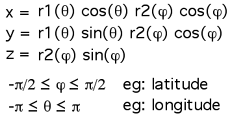The following shows the interactive interface developed to explore 3D supershapes. It is based upon X-Windows and OpenGL. It is currently available for Mac OS-X (as a UNIX application). Use of the program is straightforward, edit fields and hit return. The left mouse button rotates the model in two axes, the middle mouse button rotates about the third axis. A right mouse button brings up a menu.

• Being based upon X11 it is necessary to download and install XQuartz. While not supposedly necessary, the author suggests rebooting after installing XQuartz.

• You will need to get around the Apple security restrictions for any application not from the app store. After trying to run the program and being warned about security, go to System Preferences and Security & Privacy, in the General tab allow supershape to be opened.

• Please note that a right mouse button is required to access the menus by right clicking on the window. You can get a right mouse by either getting a 3 button USB mouse, or using the control-click on the touchpad.

 Due to the march of progress some technologies get left behind, in this case it is Apples failure to continue (correct) support for X11 on their retina displays. As such, this software is unfortunately no longer available.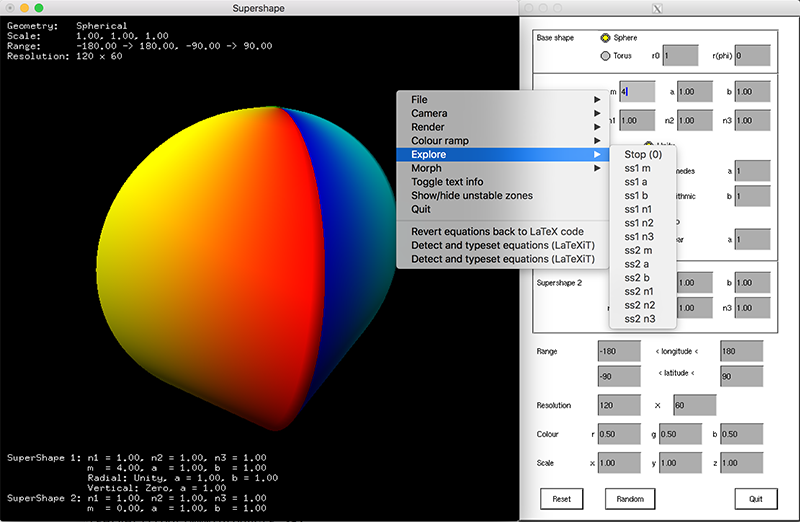Click for full size image

Command line interface

Many settings can be initialised from the command line, others control is given by single key strokes. To see a complete list type the program name at the command prompt with a "-h" option. At the time of writing these are given below.

```>supershape -h
Usage: supershape [command line options]
Command line options
-h   this text
-f   full screen
-s   active stereo
-ss   dual screen stereo
-a   auto rotate
-rw   wireframe
Mouse buttons
left   camera rotate
shift left   camera pan
middle   camera roll
shift middle   camera forward, reverse

Key Strokes
arrow keys   camera rotate
<,>   camera forward, reverse
+,-   camera zoom in, out
[,]   camera roll
r   toggle window recording
w   capture window to TGA file
a   toggle auto rotation of camera
1,2,3   different rendering mode
h   camera to home position
f1 to f6   axis aligned camera positions
ESC,q   quit
```
Features

• Exports geometry as DXF, POVRay, and LightWave (the later includes texture coordinates).

• Rendering modes include wireframe, flat shaded, and specular shading. Rendering is based upon OpenGL.

• Colour ramps can be applied to both longitude and latitude.

• Parameter space exploration by either single parameter ranges or simply choosing a random parameter set.

• A supershape can be morphed into another.

• Images can be saved to a single TGA file or repeated saving can be turned on for animations. TGA files and the naming convention used are supported by QuickTime Pro.

Examples

The 2D supershapes (but with added small thickness) can be created using this software by setting the second supershape parameters as m=0, n1=n2=n3=1, a=b=1 and using a small z axis scale factor. Indeed this can often be the preferred approach for using a 2D form within a 3D model, after all, real world objects do have some thickness. The example on the left has a thickness of 0.02 units (x and y axis dimensions of 1). Or even set the depth to 0 and vary latitude from 0 to 90 degrees as in the example on the right.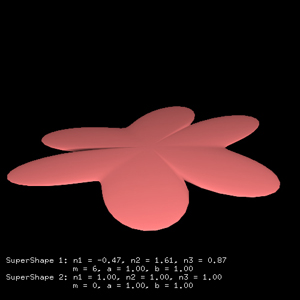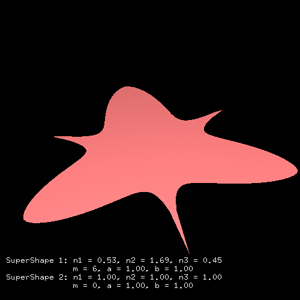Extrusions of 2D supershapes can be created by setting the second supershape parameters as m=4, a=1, b=1, and high equal values for each of n1,n2,n3. For example n1=n2=n3=200 gives relatively sharp edges.A significant portion of the parameter space results in surfaces with various types of numerical problems (powers of negative numbers, divide by 0, underflow, overflow, etc) as well as issues related to the representation of 3D graphics. The software that has been developed and created the images shown above, can display the edges where numerical problems have arisen. These regions are shown in pick as shown in the following two images.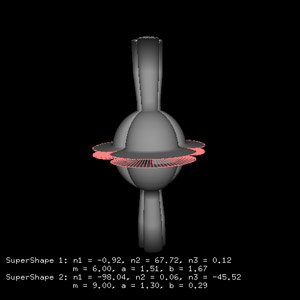The supershape function can obviously be modulated by another function. For example to create the shell like structures below, the radius (r1) of the superformula that varies the longitude is scaled by either a logarithmic or Archimedes spiral. In some cases the z coordinate is additionally made a linear function of longitude.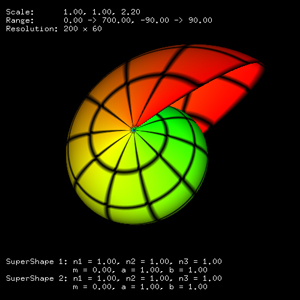In the above the two supershapes were mapped onto a topological sphere. One could map supershapes onto other forms as well, for example, a torus. In the following images the mapping is as follows: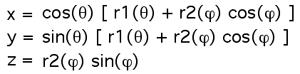# Generating the 3D Supershape in Povray

Written by Paul Bourke
July 2003

Based upon equations by Johan Gielis
Intended as a modelling framework for natural forms.

There are two (at least) ways of representing supershapes using PovRay. One is to use the built-in parametric primitive, doing it this way would result in the highest resolution/quality results unfortunately the rendering times proved to be prohibitive. The approach illustrated below is to write a macro in the PovRay scene language. The macro and an example of it's use is given in this PovRay (version 3.5) file: supershape.pov.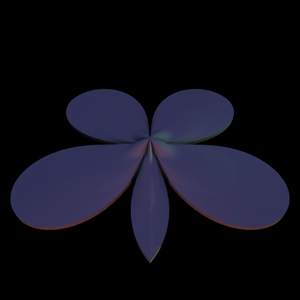SuperShape(9,1,1,92,0.3,-45,4,1,1,-0.8,88,-0.35,202)SuperShape(1,1,1,77,0.81,71.7,8,1,1,0.63,2.92,0.24,200)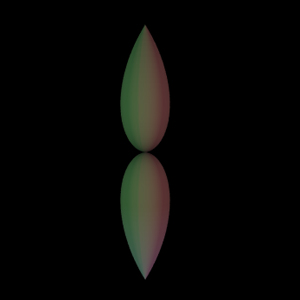SuperShape(9,1,1,-70,-0.14,77,2,1,1,0.38,4.12,-0.7,200)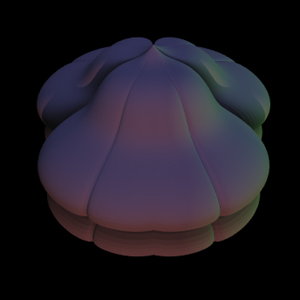SuperShape(7,1,1,20.45,-0.33,-3.54,6,1,1,-0.96,4.46,0.52,200)

Further POVRay scene examples are given below
 ss_macro1.inc example1.pov ss_macro2.inc example2.pov ss_macro3.inc example3.pov example4.pov

Contributions from the POVRay competition

 Author: SpB Title: Flying wing Source: 0001.pov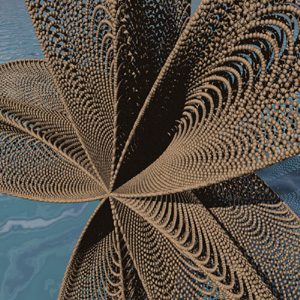Author: Dalius Dobravolskas Title: Lost in the sea Source: 0002.pov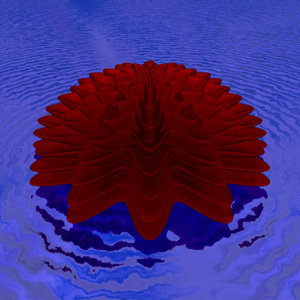Author: Paul Bourke Title: Supershape fractal Source: 0003.pov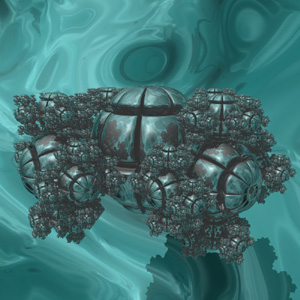Author: Stefano Tessarin Title: Flowers Source: 0004.pov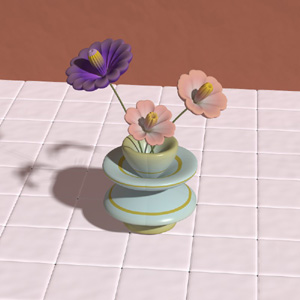Author: Emanuele Munarini Source: 0005.pov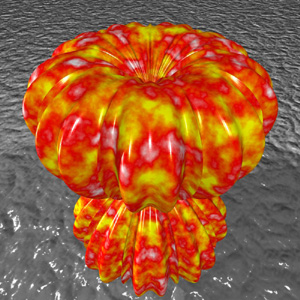Author: Emanuele Munarini Source: 0006.povAuthor: Emanuele Munarini Source: 0007.povAuthor: Emanuele Munarini Source: 0008.povAuthor: Ben Scheele Title: Butterflies find cactus flowers in a desert Source: 0009.pov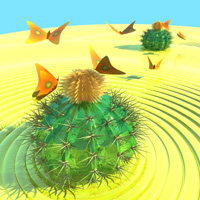Correspondence

Question: How can Superformula or Supershapes aid in data visualization? Do they help a person to gain a perspective of various data points? Are they better than pie-charts, graphs, histograms, scatter plots etc.,

Hmmm, they are kind of different things ... pie chart, graphs etc and supershapes.

In the former one takes data and uses it to control some geometry, quite simple geometry like portions of a pie, height of bars, points on a plane, lines and so on. The resulting structure is intended to provide some insight into the underlying data. At the end of the day the purpose of visualisation is to provide insight and the success of a visualisation should be judged as such.

Supershapes are (complex) 3D surfaces, they came about because Johan thought they could be used to describe a large range of biological shapes, namely leaves, flowers. The big problem with supershapes is the relationship between the variables and the final form is not always clear. So, if you gave someone a geometric form and asked what the variables are to represent that as a supershape, it is difficult, most people just play/fiddle and hope for the best.

Perhaps you are imagining mapping data to the variables and using the resulting supershape as a visualisation of the data. There may be some datasets for which this might work, but I doubt it. For the same reason, the relationship between the variables and the shape is unclear. And, if you have two very similar datasets the supershape could look very different ... not a good property for a visualisation.